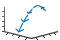# quiver3

•## 语法

``quiver3(X,Y,Z,U,V,W)``
``quiver3(Z,U,V,W)``
``quiver3(___,scale)``
``quiver3(___,LineSpec)``
``quiver3(___,LineSpec,'filled')``
``quiver3(___,Name,Value)``
``quiver3(ax,___)``
``q = quiver3(___)``

## 说明

``quiver3(X,Y,Z,U,V,W)` 在由 `X`、`Y` 和 `Z` 指定的笛卡尔坐标处，绘制具有定向分量 `U`、`V` 和 `W` 的箭头。例如，第一个箭头源于点 `X(1)`、`Y(1)` 和 `Z(1)`，根据 `U(1)` 在 x 轴方向延伸，根据 `V(1)` 在 y 轴方向延伸，并根据 `W(1)` 在 z 轴方向延伸。默认情况下，`quiver3` 函数缩放箭头长度，使其不重叠。`
````quiver3(Z,U,V,W)` 在沿曲面 `Z` 的等距点上绘制箭头，箭头的定向分量由 `U`、`V` 和 `W` 指定。 如果 `Z` 是向量，则箭头的 x 坐标范围是从 1 到 `Z` 中的元素数，并且 y 坐标均为 1。如果 `Z` 是矩阵，则箭头的 x 坐标范围是从 1 到 `Z` 中的列数，而 y 坐标范围是从 1 到 `Z` 中的行数。 ```

````quiver3(___,scale)` 调整箭头的长度： 当 `scale` 为正数时，`quiver3` 函数会自动调整箭头的长度，使其不重叠，然后将箭头长度拉伸 `scale` 倍。例如，`scale` 为 2 会使箭头长度加倍，`scale` 为 0.5 会使箭头长度减半。当 `scale` 为 `'off'` 或 `0` 时，如 `quiver3(X,Y,Z,U,V,W,'off')`，则禁用自动缩放。 ```

``quiver3(___,LineSpec)` 设置线型、标记和颜色。标记出现在由 `X`、`Y` 和 `Z` 指定的点上。如果使用 `LineSpec` 指定标记，则 `quiver3` 不显示箭尖。要指定标记并显示箭尖，请改为设置 `Marker` 属性。`
``quiver3(___,LineSpec,'filled')` 填充由 `LineSpec` 指定的标记。`
``quiver3(___,Name,Value)` 使用一个或多个名称-值对组参数指定箭头图属性。有关属性列表，请参阅 Quiver 属性。在所有其他输入参数之后指定名称-值对组参数。名称-值对组参数应用于箭头图中的所有箭头。`

``quiver3(ax,___)` 在 `ax` 指定的坐标区中而不是当前坐标区 (`gca`) 中创建箭头图。参数 `ax` 可以置于前面的语法中的任何输入参数组合之前。`

``q = quiver3(___)` 返回 `Quiver` 对象。此对象对于在创建箭头图后控制其属性非常有用。`

## 示例

```load wind X = x(5:10,20:25,6:10); Y = y(5:10,20:25,6:10); Z = z(5:10,20:25,6:10); U = u(5:10,20:25,6:10); V = v(5:10,20:25,6:10); W = w(5:10,20:25,6:10);```

```quiver3(X,Y,Z,U,V,W) axis equal``````[X,Y,Z] = sphere(10); [U,V,W] = surfnorm(X,Y,Z); quiver3(X,Y,Z,U,V,W,0) axis equal``````figure quiver3(X,Y,Z,U,V,W) axis equal``````[X,Y] = meshgrid(-2:0.25:2,-1:0.2:1); Z = X.*exp(-X.^2 - Y.^2); [U,V,W] = surfnorm(X,Y,Z);```

```quiver3(X,Y,Z,U,V,W) hold on surf(X,Y,Z) axis equal``````[X,Y] = meshgrid(-pi/2:pi/8:pi/2,-pi/2:pi/8:pi/2); Z = sin(X) + cos(Y); [U,V,W] = surfnorm(Z);```

```quiver3(X,Y,Z,U,V,W,'r') axis equal``````[X,Y] = meshgrid(-2:0.25:0,-2:0.25:0); Z1 = -0.5*(X.^2 + Y.^2); [U1,V1,W1] = surfnorm(Z1); Z2 = -X.*Y; [U2,V2,W2] = surfnorm(Z2); tiledlayout(1,2) % Left plot ax1 = nexttile; quiver3(ax1,X,Y,Z1,U1,V1,W1) axis equal title(ax1,'Left Plot') % Right plot ax2 = nexttile; quiver3(ax2,X,Y,Z2,U2,V2,W2) axis equal title(ax2,'Right Plot')``````[X,Y] = meshgrid(-3:0.5:3,-3:0.5:3); Z = 0.2*(Y.^2 - X.^2); [U,V,W] = surfnorm(Z); q = quiver3(X,Y,Z,U,V,W); axis equal q.ShowArrowHead = 'off'; q.Marker = '.';```## 输入参数

`"-"`实线`"--"`虚线`":"`点线`"-."`点划线`"o"`圆圈`"+"`加号`"*"`星号`"."``"x"`叉号`"_"`水平线条`"|"`垂直线条`"square"`方形`"diamond"`菱形`"^"`上三角`"v"`下三角`">"`右三角`"<"`左三角`"pentagram"`五角形`"hexagram"`六角形`"red"``"r"``[1 0 0]``"green"``"g"``[0 1 0]``"blue"``"b"``[0 0 1]``"cyan"` `"c"``[0 1 1]``"magenta"``"m"``[1 0 1]``"yellow"``"y"``[1 1 0]``"black"``"k"``[0 0 0]``"white"``"w"``[1 1 1]`### 名称-值参数

• `'on'` - 显示带箭尖的向量。

• `'off'` - 显示不带箭尖的向量。

• `'on'` - 缩放箭头长度以便可容纳在网格定义的坐标数据和缩放箭头内，这样它们不会发生重叠。然后，`quiver``quiver3` 函数将 `AutoScaleFactor` 应用于箭头长度。

• `'off'` - 不缩放箭头长度。